Function Repository Resource:

# FocalLossLayer

A NetGraph layer implementing focal loss

Contributed by: Michael Sollami
 ResourceFunction["FocalLossLayer"][α,γ] represents a net layer that implements focal loss.

## Details and Options

ResourceFunction["FocalLossLayer"] exposes the following ports for use in NetGraph etc.:
 "Input" scalar values between 0 and 1, or arrays of these "Target" scalar values between 0 and 1, or arrays of these "Loss" real number
ResourceFunction["FocalLossLayer"] is a variant of binary cross-entropy (see CrossEntropyLossLayer) that downweights well-classified examples.
ResourceFunction["FocalLossLayer"] is defined in the 2017 paper "Focal Loss for Dense Object Detection".
The paper's authors suggest default values of 0.25 and 2 for α and γ, respectively.
ResourceFunction["FocalLossLayer"] takes the same options as NetGraph.

## Examples

### Basic Examples (4)

Create a focal loss layer:

 In:=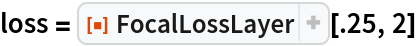Out=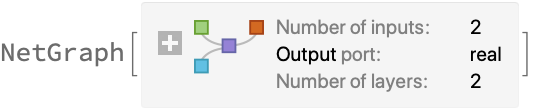Apply it to a given input and target:

 In:=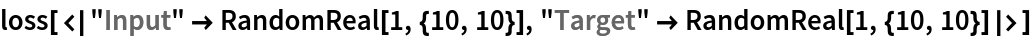Out=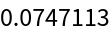Use FocalLossLayer with single probabilities for the input and target:

 In:=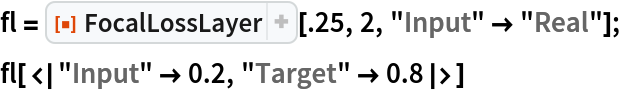Out=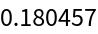Apply FocalLossLayer with inputs and targets being matrices of binary-class probabilities:

 In:=Out=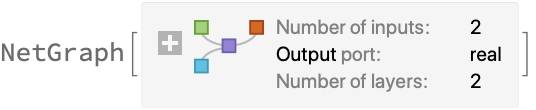Apply the layer to an input and target:

 In:=Out=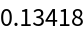Use a focal loss layer during training:

 In:=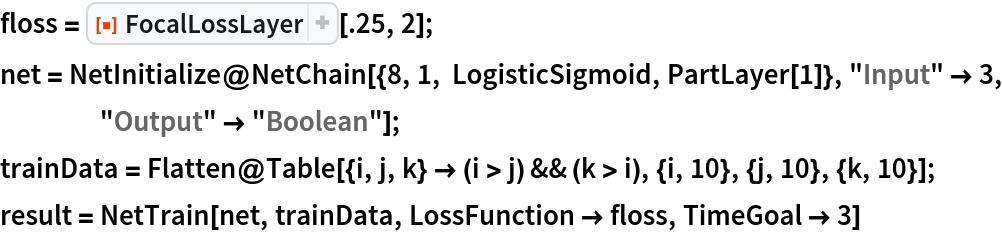Out=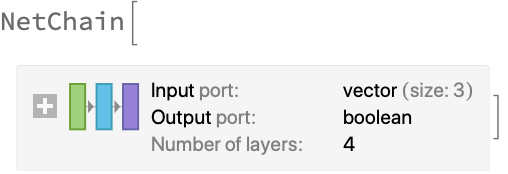In:=Out=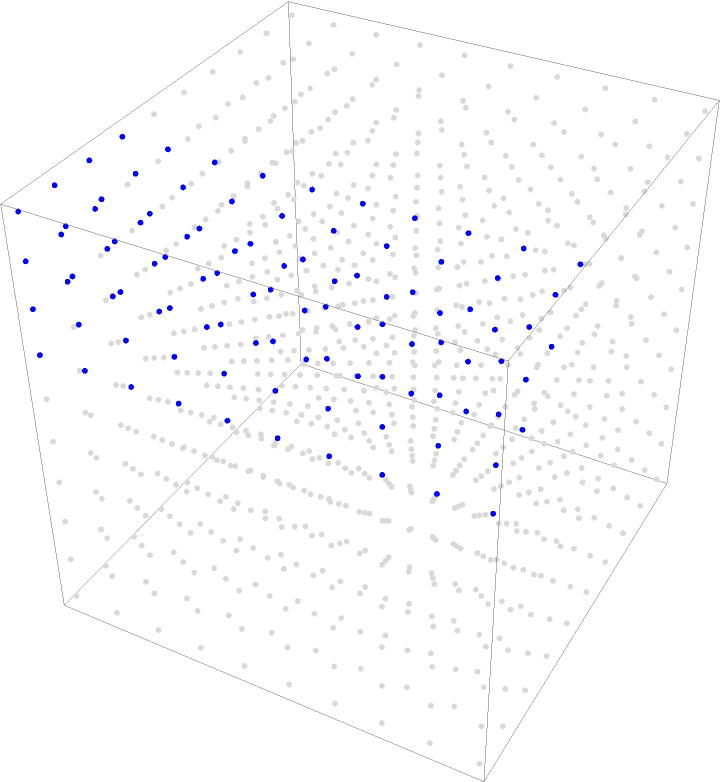### Scope (1)

Define a FocalLossLayer operating on color images:

 In:=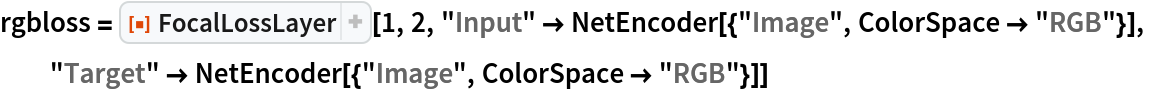Out=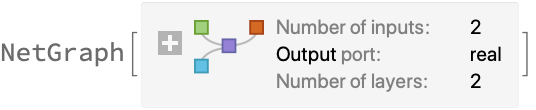In:=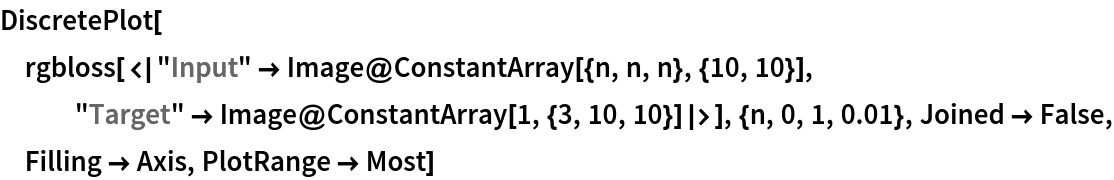Out=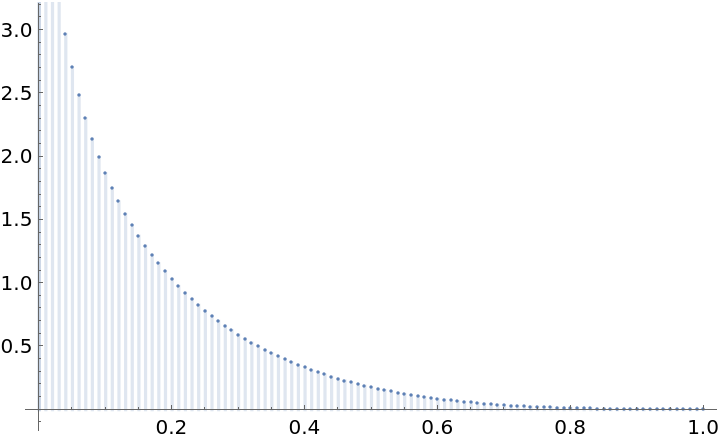### Properties and Relations (1)

Plot a comparison of FocalLossLayer (abbreviated as "FL") and standard CrossEntropy (abbreviated as "CE"). Note that setting γ>0 reduces the relative loss for well-classified examples (pt>.5), thus focusing on the harder examples:

 In:=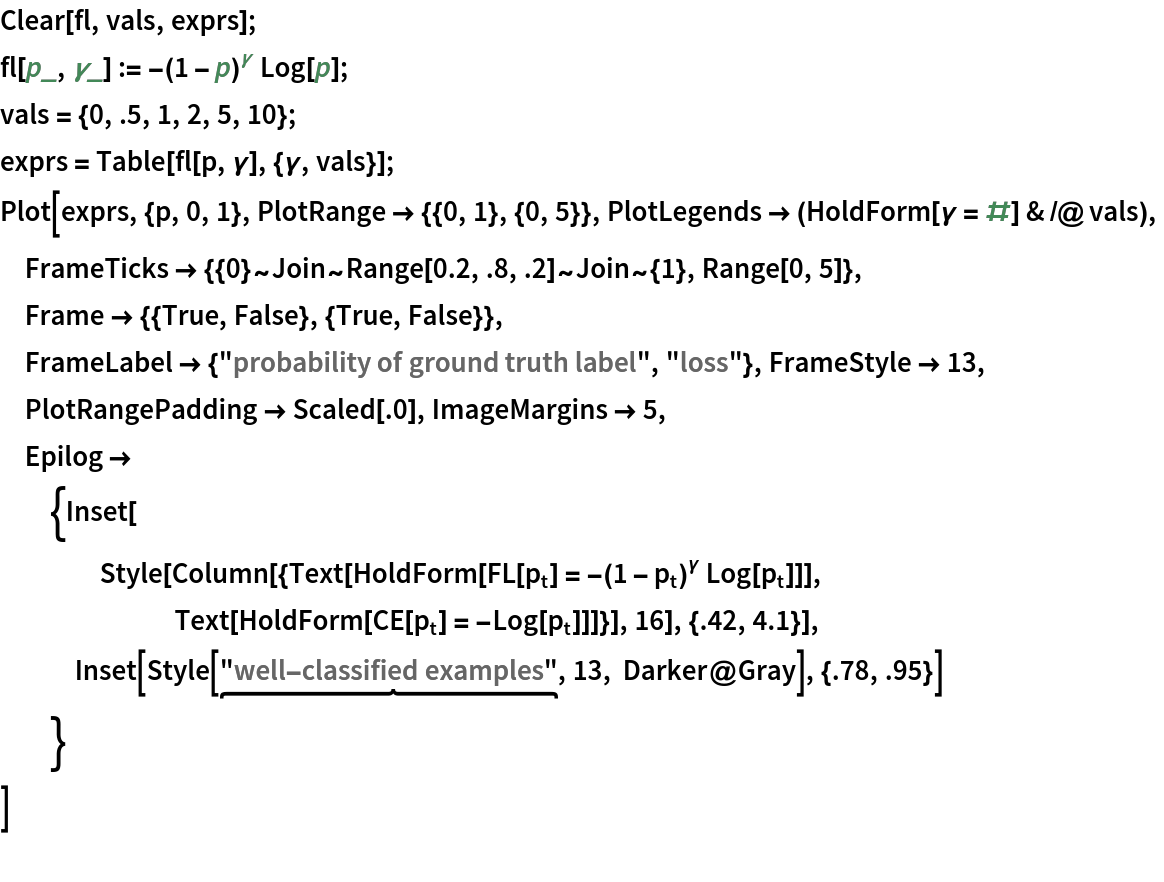Out=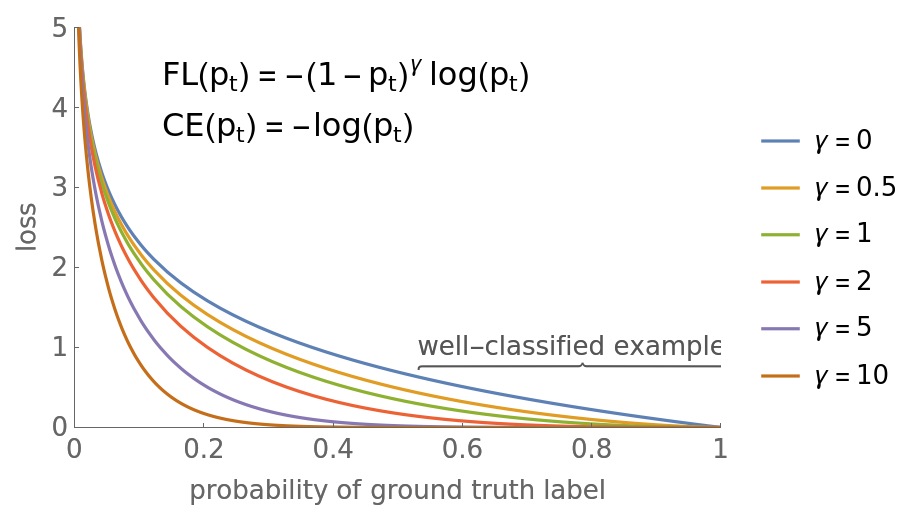Michael Sollami

## Version History

• 1.0.0 – 27 January 2020